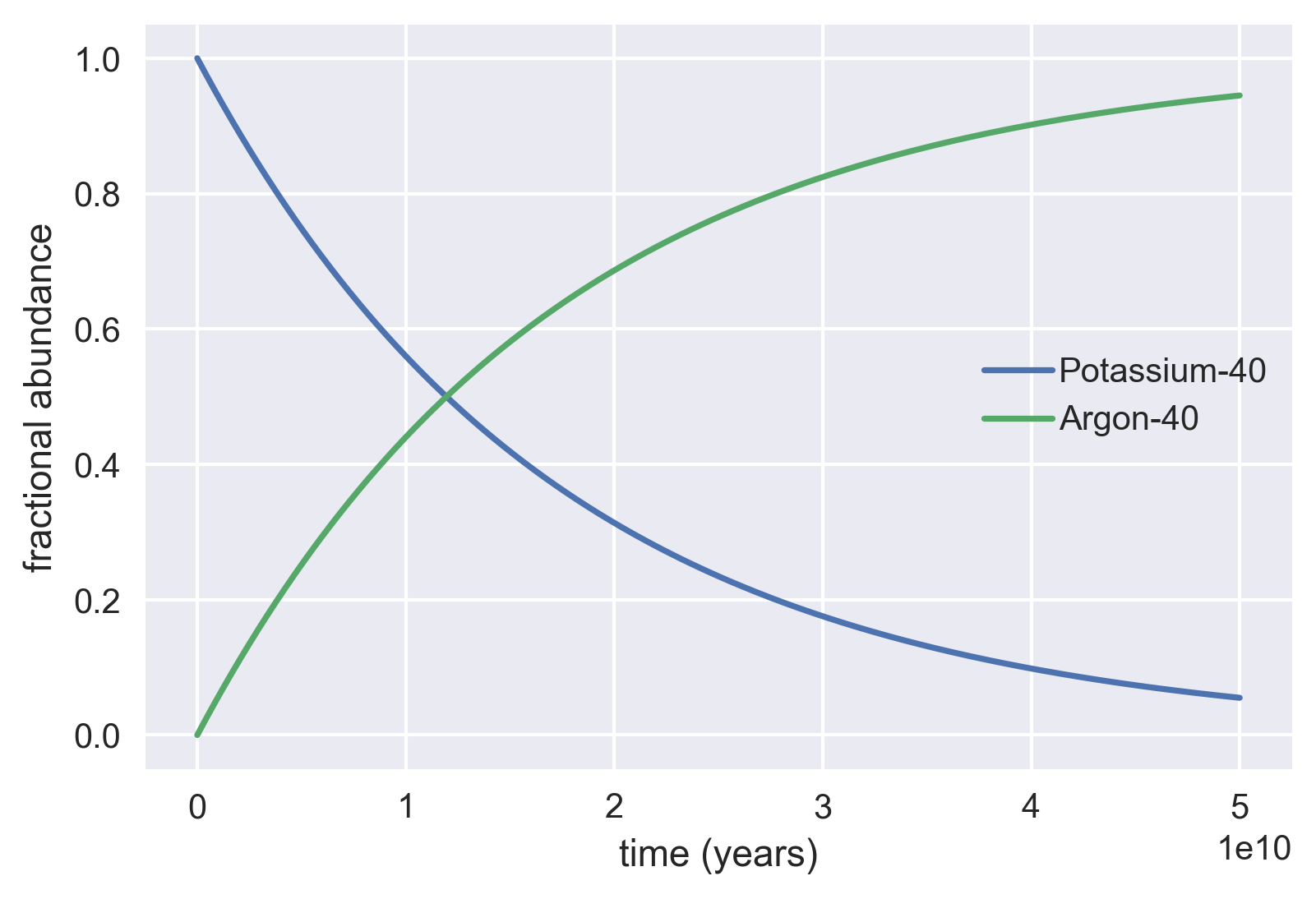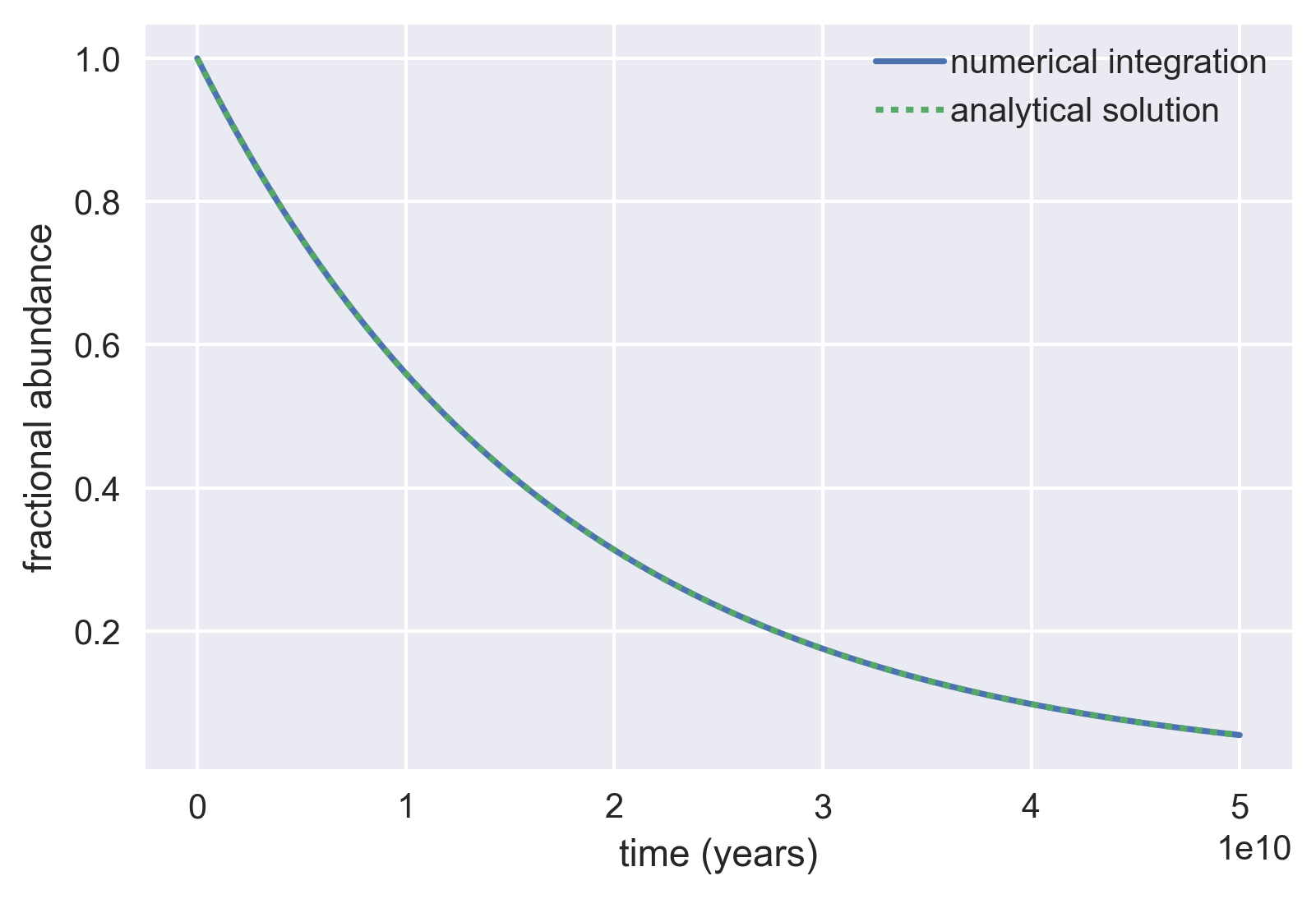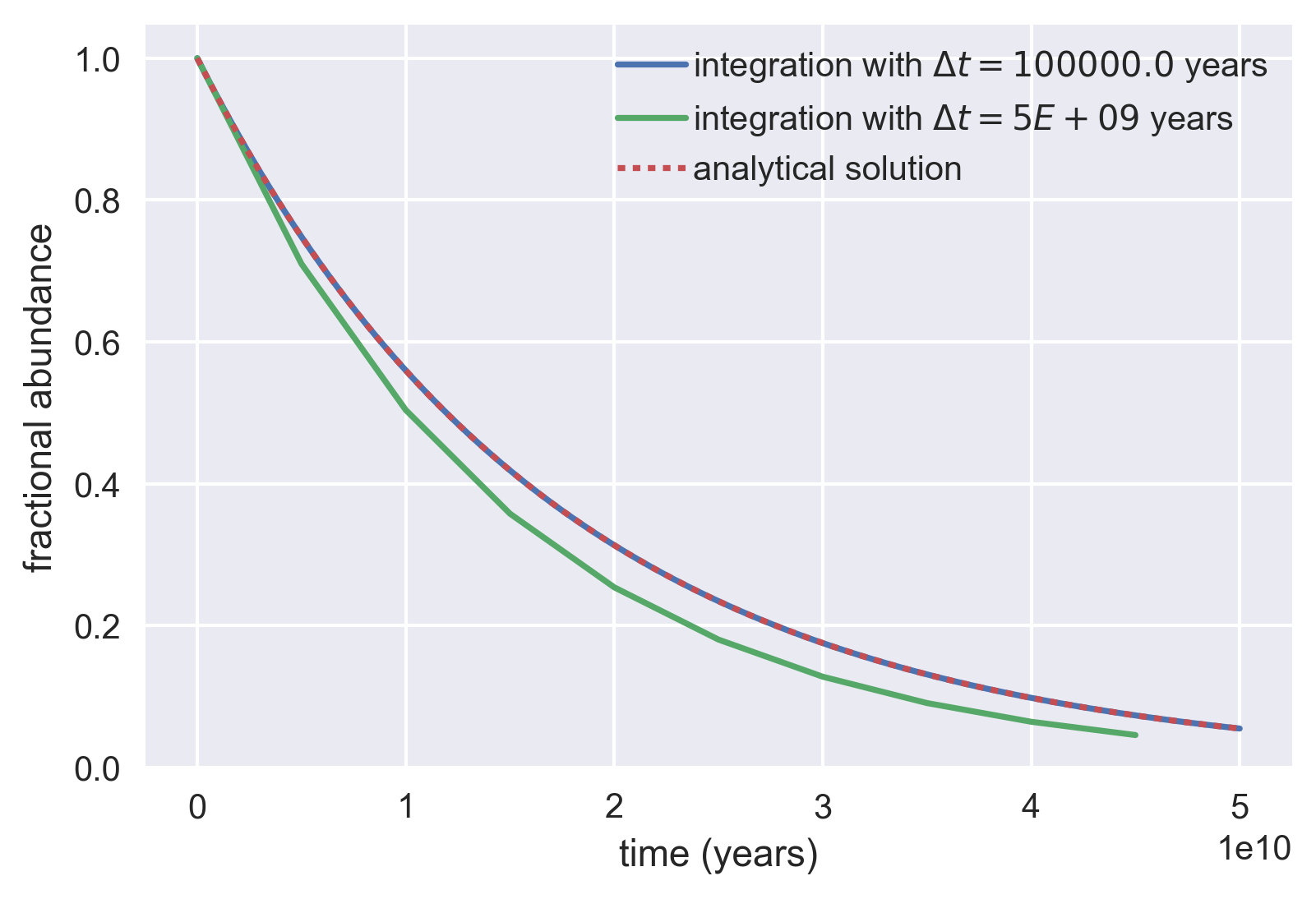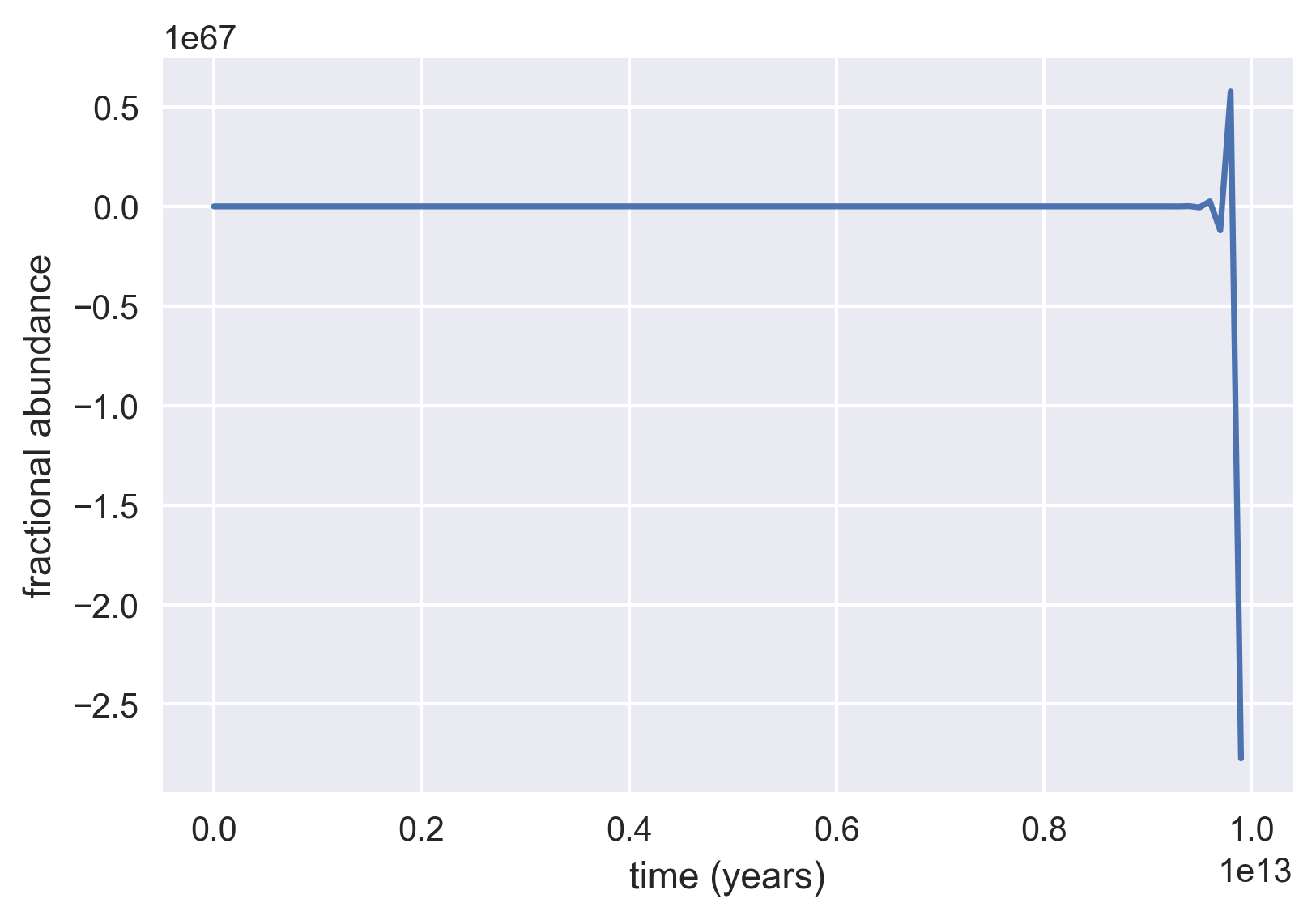# Tutorial 2: Numerical Integration¶

In :
import numpy as np
import matplotlib.pyplot as plt
import seaborn as sns
%matplotlib inline


In this tutorial, we will cover how to write a simple numerical integrator using the Euler Forward method and examine the dynamics of radioactive decay.

## The Forward Euler Method¶

Developing simple ways to solve ordinary differential equations has long been an area of intense research. While deriving the analytical solution may be simple in some cases, it is often useful to solve them numerically, especially if slamming out the analytical solution will give you carpal tunnel.

While there are many ways to numerically integrate these equations, in this tutorial we will examine the Forward Euler method. Say we have an ordinary differential equation such as

$${dx \over dt} = -{x \over \tau} \tag{1}$$

where $\tau$ is some constant and $t$ is time. Rather than solving this analytically (although it is trivial), we can solve it numerically by starting at some given value of $x$, evaulating Equation 1 for a given time step $\Delta t$, and updating the new value of $x$ at this new time $t + \Delta t$. We can phrase this mathematically as

$$x(t + \Delta t) = x(t) - {x(t) \over \tau} \Delta t.\tag{2}$$

Say our initial value ($x$ at $t=0$) is $x = 10$ and $\tau=1$. We can take a time step $\Delta t=0.1$ and find that the change in value of $x$ is

$$\Delta x=-{x \over \tau} \Delta t = -1. \tag{3}$$

We can then compute the new value of $x$ at time $t + \Delta t$ as

$$x(t + \Delta t) = x(t) + \Delta x = 10 - 1 = 9. \tag{4}$$

We can then take another step forward in time and repeat the process for as long as we would like. As the total time we'd like to integrate over becomes large, it becomes obvious why using a computer is a far more attractive approach than scribbling it by hand.

A major point to be wary of is the instability of this method. The error in this scales with the square of our step size. We must choose a sufficiently small step in time such that at most only one computable event must occur. For example, if we are integrating exponential growth of bacterial cells, we don't want to take time steps larger than a cell division! This requirement is known as the Courant-Friedrichs-Lewy condition and is important for many different time-marching computer simulations.

As is often the case, the best way to learn is to do. Let's give our digits some exercise and numerically integrate a familiar old friend $-$ radioactive decay

## Radioactive Decay In Gale Crater¶

### More than just a Sci-Fi trope¶

As you probably learned in high school (or in your thorough study of mid-century sci-fi film), unstable elemental isotopes can undergo radioactive decay changing their identity to that of a different element coupled with the emission of another particle (such as an electron, gamma ray, or alpha particle).

As you will learn later on in the course, this property of unstable isotopes can be used to infer the age of a variety of objects. The most famous example of this is the use of Carbon-14 abundance to date objects of organic origin, although radiometric dating has many different uses. In this tutorial, we'll consider one of these other applications. Potassium-Argon dating is a method of radiometric dating which uses the fractional abundance of argon trapped in minerals and other materials as a measure of the age of the object. Being a noble gas, argon is remarkably inert and is typically found in its elemental state. However, a variety of minerals have Argon coordinated in the crystal lattice. This results from the decay of Potassium-40 into Argon-40. One this decay occurs, the newly formed Argon-40 remains trapped in the crystal lattice.

Potassium-40 has a very long half-life of 1.25 $\,\times\,$ 10$^9$ years meaning that it can be used to find the absolute age of objects older than 100,000 years. In fact, this method of dating was used by the Curiosity Rover on Mars in 2013 to confirm age of rocks in Gale crater to be between 3.86 and 4.56 billion years old.

### Numerical integration of radioactive decay.¶

For this example, let's imagine we have large rock sample from Gale Crater sitting in the middle of Gale Crater. This sample of rock has 1 million Potassium-40 atoms coordinated within it. Our question is, how will the fractional abundace of these Potassium isotopes change as a function of time?

We know that the decay time of one given Potassium-40 atom is independent of all other atoms in the sample, meaning that the decay will be constant. We can write the ordinary differential equation for this decay as

$${dN \over dt} = -\lambda N \tag{5},$$

where $N$ is the number of radioactive nuclei, $t$ is the time, and $\lambda$ is the decay constant in units of inverse time.

We can write this in a similar manner as Equation 3 using the Forward Euler method to obtain

$$N(t + \Delta t) = N(t) - \lambda N(t) \Delta t \tag{6},$$

where $N(t)$ is the number of Potassium-40 isotopes at time (t) and $\Delta t$ is a small step forward in time.

While we can solve this ODE analytically to obtain the equation for exponential decay, let's integrate Equation 5 using this method. Before we perform the integration, we'll need to define a few variables.

In :
# Define a few parameters for the integration
n_0 = 1E6 # Initial number of Potassium-40 nuclei
lam =  5.8E-11 # Decay constant in units of inverse years.
total_time = 5E10 # Total time to run the integration in units of years.


In the above code cell, we defined the number of Potassium-40 atoms in our rock sample, the decay time, and the total time over which we would like to integrate (50 billion years!).

Notice that we defined the decay constant $\lambda$ as lam and not lambda. This is because lambda is actually something called an anonymous function in Python. Because of the way this anonymous function generator is constructed, Python will yell at you with error messages if you try to name a variable lambda!

We have one last parameter left to define $-\, \Delta t$. As noted in the beginning section of this tutorial, we have to be careful with the time step when performing our integration. We want to take steps that are sufficiently short such that no more than one event will occur during our time step. Since Potassium-40 has a decay time of 5.5 $\times$ 10$^{-11}$ per year (for the Potassium-40 to Argon-40 transition), we can take time steps of 10$^5$ years and feel comfortable that we are taking many steps per event rate. We also need to define a vector in which we will keep track of the number of Potassium-40 nuclei remaining in our sample and set the initial condition of 1 million atoms.

In :
# Define the time step for the integration.
dt = 1E5

# Set up the time vector. We'll start from 0 going to the total time, taking
# steps of dt. For this, we will use the arange function in numpy.
time_range = np.arange(0, total_time, dt)

# Set up the storage vector to keep track of the number of Potassium-40 nuclei
n_t = np.zeros(len(time_range))

# Set the initial condition.
n_t = n_0


We are now ready to begin the integration. To do this, we will set up a loop ranging from the very first time point all the way up to the last time point. We'll have to set up our range in our loop such that we can compute $N(t + \Delta t)$ even if we are at the last time point.

In :
# Begin the integration. Go from 0 to the total length - 1 so we can still
# compute the n_t[i + 1] value.
for i in range(len(time_range) - 1):

# Compute the change in the number of Potassium-40 nuclei
dN = -lam * n_t[i] * dt

# Update the current number in the storage vector.
n_t[i + 1] = n_t[i] + dN


And we are finished! In just three lines of code, we were able to do a numerical integration of an ODE at 500,000 time points. Let's plot the fractional abundance of the Potassium-40 as well as the abundance of Argon-40 in our sample as a function of time.

In :
# Convert our n_t to fractional abundance.
K40_frac = n_t / n_0
Ar40_frac = 1 - K40_frac

# Plot our integration.
plt.plot(time_range, K40_frac, '-', label='Potassium-40')
plt.plot(time_range, Ar40_frac, '-', label='Argon-40')
plt.xlabel('time (years)')
plt.ylabel('fractional abundance')
plt.legend()

Out:
<matplotlib.legend.Legend at 0x1160c4358>Nice! Just as we expect, the number of non-decayed particles decreases exponentially with time. We can see that in about 50 billion years, there will only be about a tenth of the original Potassium-40 remaining in our rock sample (don't plan on verifying this experimentally).

As was mentioned in the first section of this tutorial, Forward Euler integration is an unstable algorithm and can be inaccurate. How well does our numerical integration match the analytical solution?

We can solve Equation 5 analytically to produce

$$N(t) = N_0e^{-\lambda t}$$

where $N_0$ is the initial number of Potassium-40 atoms in our sample. Let's plot the analytical solution and our numerical integration to see how well they agree.

In :
# Evaluate the analytical solution.
analytic_soln = n_0 * np.exp(-lam * time_range)
analytic_frac = analytic_soln / n_0

# Plot our numerical integration.
plt.plot(time_range, K40_frac, '-', label='numerical integration')

# Plot the analytical solution as a dotted line.
plt.plot(time_range, analytic_frac, ':', label='analytical solution')
plt.ylabel('fractional abundance')
plt.xlabel('time (years)')
plt.legend()

Out:
<matplotlib.legend.Legend at 0x117902d68>That seems to be dead-on the same! This is due to the simplicity of this ODE coupled with the fact we took a short enough time step. To be instructive, let's increase our time step and see how much the numerical integration and the analytical solution agree. We'll increase our time step by four and a half orders of magnitude to 5 billion years.

In :
# Define the large time step.
bigstep = 5E9
timerange_bigstep = np.arange(0, total_time, bigstep)

# Perform the integration.
nt_bigstep = np.zeros(len(timerange_bigstep))
nt_bigstep = n_0
for i in range(len(timerange_bigstep) - 1):
dN = -lam * nt_bigstep[i] * bigstep
nt_bigstep[i + 1] = nt_bigstep[i] + dN

# Convert the numbers to fractional abundance.
K40_frac_bigstep = nt_bigstep / n_0


Let's plot the analytical solution and both of our integrations on the same axes.

In :
# Plot the solutions
plt.plot(time_range, K40_frac, '-', label='integration with $\Delta t = %s$ years'
%dt)
plt.plot(timerange_bigstep, K40_frac_bigstep, '-',
label='integration with $\Delta t= %G$ years' %bigstep)
plt.plot(time_range, analytic_frac, ':', label='analytical solution')

# Add axes labels and a legend.
plt.xlabel('time (years)')
plt.ylabel('fractional abundance')
# plt.xlim([0.8E11, 1E11])
# plt.ylim([-.2, 0.2])
plt.legend()

Out:
<matplotlib.legend.Legend at 0x1243faba8>In the above code cell, we used another magic string formatter %G. This forces long numbers which are converted to strings to use scientific notation. As a refresher, you can see the documentation for more details on more advanced string formatting.

Now we can see why taking an appropriate time step is so important. Even though our time step was smaller that the inverse decay time, it was relatively close, meaning that more than one event occurring per time step was not neglibile. In fact, if we let this integration run for an even longer period of time, the instability in the method will become very pronounced! For that reason, it's often better to take very small time steps relative to the rate of change of the system of interest. The smaller the step you take, however, will increase the time it takes to compute.

In :
# Let the large time step integration run even further.
long_time = 1E13
bigstep = 1E11  # Make an even bigger step
timerange_bigstep = np.arange(0, long_time, bigstep)

# Perform the integration.
nt_bigstep = np.zeros(len(timerange_bigstep))
nt_bigstep = n_0
for i in range(len(timerange_bigstep) - 1):
dN = -lam * nt_bigstep[i] * bigstep
nt_bigstep[i + 1] = nt_bigstep[i] + dN

# Convert the numbers to fractional abundance.
K40_frac_bigstep = nt_bigstep / n_0

# Plot it!
plt.plot(timerange_bigstep, K40_frac_bigstep, '-')
plt.xlabel('time (years)')
plt.ylabel('fractional abundance')

Out:
<matplotlib.text.Text at 0x11791dcc0>At very long integration times, the instability with this large step size is very noticeable as we start stepping above and below our lower bound! For this reason, it's often better to take very small time steps relative to the rate of change of the system of interest. The smaller the step you take, however, the longer you will need to compute!

## In summary...¶

In this tutorial, we've learned how to make our computers do the work for numerically solving ordinary differential equations. While this is a useful tool pedagogically, there are more sophisticated and robust methods available for numerical integration.